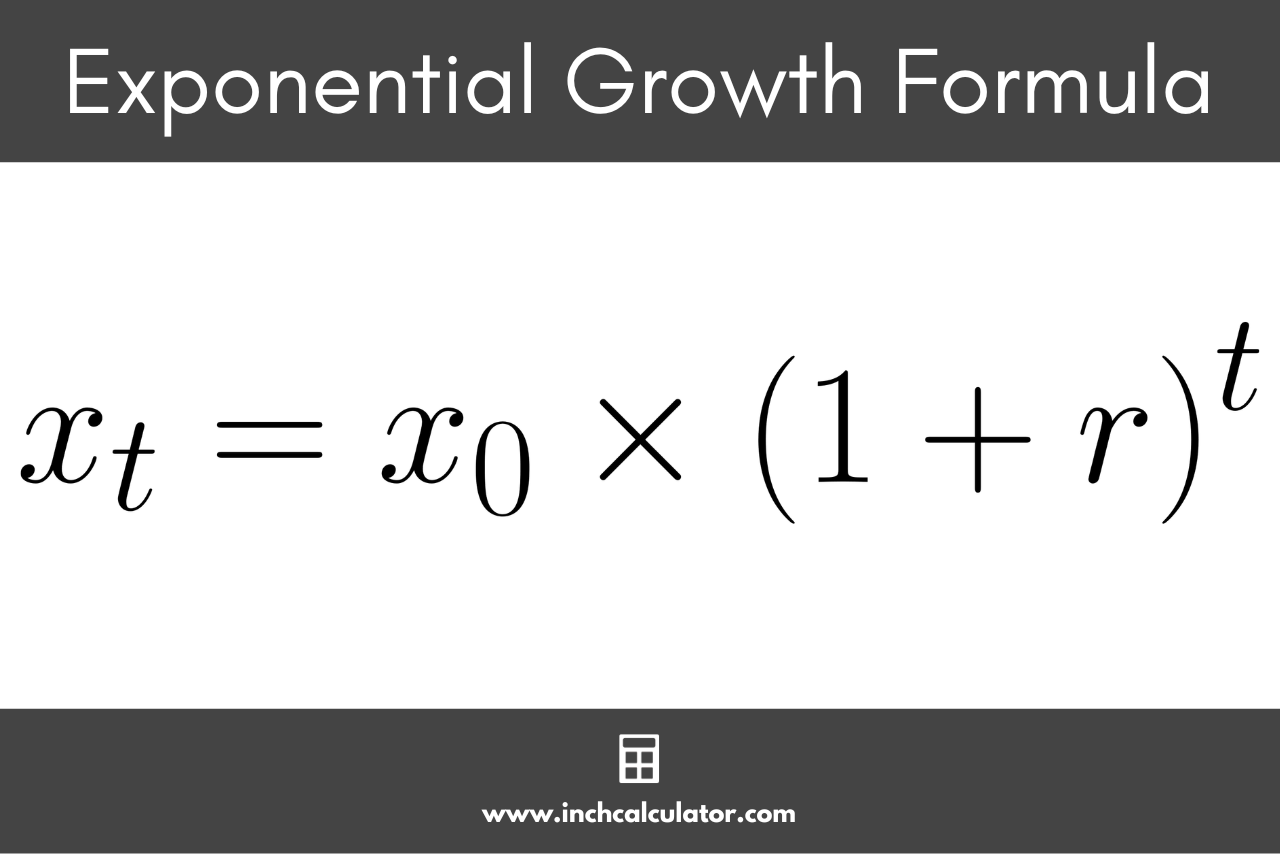# Exponential Growth Calculator

Use our exponential growth calculator to find the final value, initial value, growth rate, or time.

Calculate:
x_{t}=x_{0} \times \left ( 1 + r \right ) ^{t}
%
x_{t}=x_{0} \times \left ( 1 + r \right ) ^{t}
%
x_{t}=x_{0} \times \left ( 1 + r \right ) ^{t}
x_{t}=x_{0} \times \left ( 1 + r \right ) ^{t}
%

## Final Value:

xt =

Learn how we calculated this below

## How to Calculate Exponential Growth

Exponential growth is the growth of a value by the same percentage over a number of time periods, where the percentage of growth during each period includes the value of growth from the previous periods.

Exponential growth is the mechanism behind things such as compound interest and half-life decay.

You can calculate the amount of growth in a value using the exponential growth formula.

### Exponential Growth Formula

The formula to find a value using the exponential growth formula is:

x_{t}=x_{0} \times \left ( 1 + r \right ) ^{t}

The final value xt is equal to the initial value x0 times 1 plus the growth rate r raised to the power of the number of time periods t.By using a negative growth rate, you can also calculate the amount of exponential decay in a value.

For example, let’s calculate the growth in a savings account containing $100 that grows at a rate of 7% over 20 years. x_{t}=\$100 \times \left ( 1 + 7\%/100 \right ) ^{20}
x_{t}=\$100 \times 1.07^{20} x_{t}=\$386.97

So, a $100 savings account that grows exponentially at a rate of 7% per year over 20 years will have a final value of$386.97.